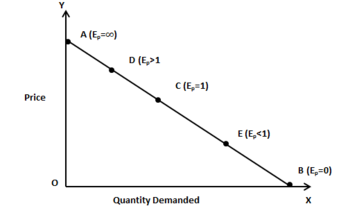# Point MethodThe point method of measurement of price elasticity demand is also known as geometric method or graphical method. This method was developed by Alfred Marshall. This method is used to measure price elasticity of demand at any given point in the demand curve. This method is suitable when a change in price and the consequent change in quantity demanded is very small.
It can be expressed as :The price elasticity of demand on a demand curve is different at its different points. The figure shows the different price elasticity of demand at different points along the same demand curve-In this above figure X-axis represents quantity demanded and Y-axis represents price of the commodity. The downward slopping curve AB represents linear demand curve. If we suppose C as the middle point of the demand curve AB. Using the formula  of point elasticity of demand given above , we can find out coefficient of price elasticity of demand at different points of the demand curve by using point method as follows:Thus, using the formula of point method, we can calculate the price elasticity of demand at different points of the demand curve.